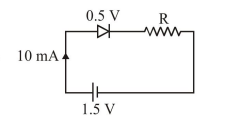# When a diode is forward biased, it has a voltage drop of 0.5 V.

Question:

When a diode is forward biased, it has a voltage drop of $0.5 \mathrm{~V}$. The safe limit of current through the diode is $10 \mathrm{~mA}$. If a battery of emf $1.5 \mathrm{~V}$ is used in the circuit, the value of minimum resistance to be connected in series with the diode so that the current does not exceed the safe limit is :

1. $100 \Omega$

2. $50 \Omega$

3. $300 \Omega$

4. $200 \Omega$

Correct Option: 1

Solution:$1.5-0.5-\mathrm{R} \times 10 \times 10^{-3}=0$

$\therefore \mathrm{R}=100 \Omega$